﻿ 基于分形自回归模型的广东省旅游人数预测 Predicting Tourist Number of Guangdong Province Based on Fractal Auto-Regressive Model

Statistics and Application
Vol. 08  No. 01 ( 2019 ), Article ID: 28903 , 6 pages
10.12677/SA.2019.81016

Predicting Tourist Number of Guangdong Province Based on Fractal Auto-Regressive Model

Huan Zhao, Weidong Fang

School of Mathematics, South China University of Technology, Guangzhou Guangdong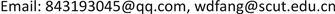Received: Jan. 27th, 2019; accepted: Feb. 11th, 2019; published: Feb. 19th, 2019ABSTRACT

An effective prediction on tourist number is good for government and related departments taking steps timely to cope with passenger flows. At the same time, it can provide proposal for development, planning and management of local economy. This article adopts monthly data of tourist number coming to Guangdong Province from 2001 to 2017, takes advantage of its long memory, establishes ARFIMA model, and separately predicts twelve months’ tourist numbers in 2017. The result shows that the prediction is effective by comparing true value with predicted value.

Keywords:Long Memory, ARFIMA Model, Fractional-Order Difference, Predict1. 引言

2. ARFIMA模型及预测

2.1. ARFIMA模型定义

2.2. ARFIMA模型建立

ARFIMA模型建立具体步骤如下：

2) 数据预处理及纯随机性检验：对表现非平稳的序列清理其中的波动趋势，使之平稳；对表现平稳的序列对其进行纯随机性检验。

3) 判断序列的长记忆性及清除短记忆因素：对得到的平稳非白噪声序列，画出自相关图，若函数图象呈多项式速度缓慢递减，在滞后阶数很大时仍有较强的自相关性，表明序列具有长记忆性。通过建立自回归AR(P)模型，清除短影响因素。

4) 分数阶差分：通过R/S分析法计算长记忆性的平稳非白噪声序列的Hurst指数，利用 $d=H-0.5$ 求出分数阶差分的数值，对序列进行分数阶差分。

5) ARFIMA模型定阶：对分数阶差分后的序列建立ARMA(p,q)模型，确定p，q的值，即得ARFIMA(p,d,q)。

6) 估计模型中未知参数 ${\varphi }_{1},{\varphi }_{2},\cdots ,{\varphi }_{p},{\theta }_{1},{\theta }_{2},\cdots ,{\theta }_{q}$ 的值。

7) 检验模型有效性并进行预测。

2.3. ARFIMA模型预测

1) 画出序列 $\left\{{X}_{t}\right\}$ 的时序图以及自相关图，图1是时序图。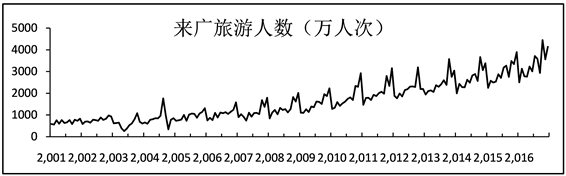Figure 1. Traveling number sequence diagram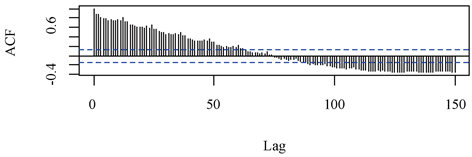Figure 2. Traveling number autocorrelogram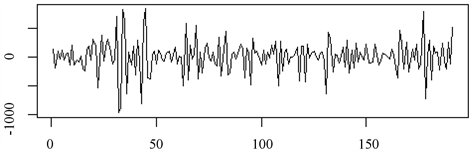Figure 3. The sequence diagram after the first difference and the first seasonal difference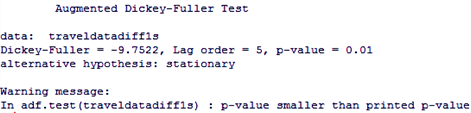P值是小于0.05，因此可以判定序列平稳。

2) 对 $\left\{{Y}_{t}\right\}$ 进行纯随机性检验，计算得出在不同滞后阶数下，Q检验统计量的P值均远小于0.05，因而是平稳非白噪声序列，且蕴含的值得提取的信息。

3) 对 $\left\{{Y}_{t}\right\}$ 建立自回归AR(P)模型，清除短影响因素，根据AIC最小信息量准则选定P = 12，模型参数分别为−0.6185，−0.6730，−0.4458，−0.4331，−0.2784，−0.3071，−0.1013，−0.1086，−0.0394，0.1071，0.1742，−0.1343，利用AR(12)估计的值建立置信水平为95%的置信区间，删除掉 $\left\{{Y}_{t}\right\}$ 中35个奇异值，得到新的序列 $\left\{{Z}_{t}\right\}$ ，过程如图5所示。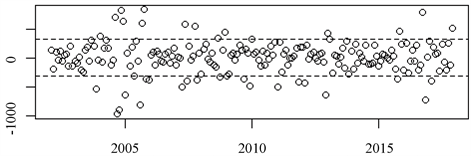Figure 5. Clear short memory factor sequence diagram

4) 计算分数阶差分d：运用修正的R/S分析法计算得到Hurst  指数为0.5788384，根据公式

$d=H-0.5$ ，即得 $d=0.0788384$ ，将分数差分后的序列记为 $\left\{{Z}_{t}\right\}$ ，则有 ${Z}_{t}={\left(1-L\right)}^{d}{Y}_{t}$ ，且满足 $\phi \left(L\right){Z}_{t}=\theta \left(L\right){a}_{t}$ ，是均值为0的ARMA(p,q)序列。

$\left\{{Z}_{t}\right\}$ 建立ARMA模型，根据自相关和偏自相关图的滞后阶数大致确定p，q范围，再利用AIC准则，选择使其值最小对应的模型为ARFIMA(1,0.0788384,0)，不同模型AIC值如表1所示。

5) 利用R软件可得模型的参数方程为 ${Z}_{t}=22.00392-0.35{Z}_{t-1}+{\epsilon }_{t}$

$\left(1+0.35L\right){\left(1-L\right)}^{0.0788384}\left(1-{L}^{12}\right){\nabla }^{1}{X}_{t}=22.00392+{\epsilon }_{t}$ ，其中 ${\epsilon }_{t}~N\left(0,20728\right)$

6) 对模型的残差进行显著性检验，得到在各阶延迟下的统计量LB统计量t的P值都远大于0.05，对系数进行显著性检验，结果表明系数显著非0，即模型拟合有效，利用模型ARFIMA(1,0.0788384,0)对2017年来广的旅游人数进行预测，并与真实值进行比较，如表2所示。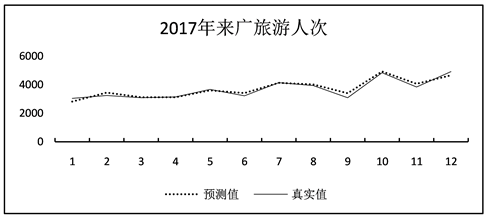Figure 6. Autocorrelation function of agriculture and forestry’s index

3. 结语

Predicting Tourist Number of Guangdong Province Based on Fractal Auto-Regressive Model[J]. 统计学与应用, 2019, 08(01): 143-148. https://doi.org/10.12677/SA.2019.81016

1. 1. Granger, C.W.J. and Joyeux, R. (1980) An Introduction to Long-memory Time Series Models and Fractional Differ-encing. Journal of Time Series Analysis, 1, 15-29. https://doi.org/10.1111/j.1467-9892.1980.tb00297.x

2. 2. Granger, C.W.J. (1980) Long Memory Relationships and the Aggregation of Dynamic Models. Journal of Econometrics, 14, 227-238. https://doi.org/10.1016/0304-4076(80)90092-5

3. 3. 冯春山, 吴家春, 蒋馥. 石油价格的ARFIMA模型预测研究[J]. 上海理工大学学报, 2005, 27(6): 539-542.

4. 4. 姚瑾. 应用ARFIMA模型对金融时间序列长期记忆性的研究[D]: [硕士学位论文]. 沈阳: 东北大学, 2006.

5. 5. 翁钢民, 郑竹叶, 刘洋. 我国入境旅游预测: 基于ARFIMA模型的研究[J]. 商业研究, 2009(6): 1-4.

6. 6. 王振寰, 孙鹏哲, 陶伟庆, 邓茗予, 杨婷. 基于ARFIMA模型的内蒙古农林牧渔业总产值研究[J]. 内蒙古师范大学学报(自然科学汉文版), 2014(6): 696-698.

7. 7. 刘凯, 张晞. 基于分数阶差分ARIMA模型的煤炭消费预测[J]. 矿业科学学报, 2017(5): 85-92.

8. 8. 陈鹏, 李星野. 基于ARFIMA-GARCH模型族的黄金价格预测分析[J]. 电子商务, 2018(5): 42-44.

9. 9. 广东省统计信息网[EB/OL]. http://www.gdstats.gov.cn/tjsj/lyy/.

10. 10. Pai, J.S. and Ravishanker, N. (1996) Bayesian Modelling of ARFIMA Processes by Markov Chain Monte Carlo Methods. Journal of Forecasting, 15, 63-82. https://doi.org/10.1002/(SICI)1099-131X(199603)15:2<63::AID-FOR606>3.0.CO;2-5